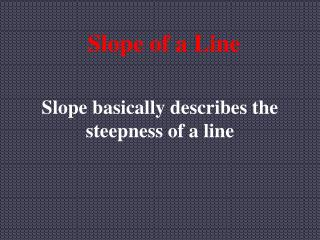DownloadDownload PresentationSlope of a Line

# Slope of a Line

Télécharger la présentation## Slope of a Line

- - - - - - - - - - - - - - - - - - - - - - - - - - - E N D - - - - - - - - - - - - - - - - - - - - - - - - - - -
##### Presentation Transcript

1. Slope of a Line Slope basically describes the steepness of a line

2. If a line goes up from left to right, then the slope has to be positive Conversely, if a line goes down from left to right, then the slope has to be negative

3. Definitions of Slope Slope is simply the change in the vertical distance over the change in the horizontal distance

4. The formula above is the one which we will use to find the slope of specific lines In order to use that formula we need to know, or be able to find 2 points on the line

5. If a line is in the form Ax + By = C, we can use the following formula to find the slope:

6. Examples

7. Horizontal lines have a slope of zero while vertical lines have no slope m = 0 Vertical Horizontal m = no slope

8. This powerpoint was kindly donated to www.worldofteaching.com http://www.worldofteaching.com is home to over a thousand powerpoints submitted by teachers. This is a completely free site and requires no registration. Please visit and I hope it will help in your teaching.